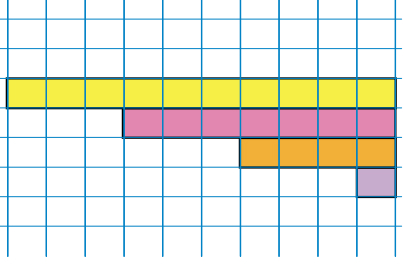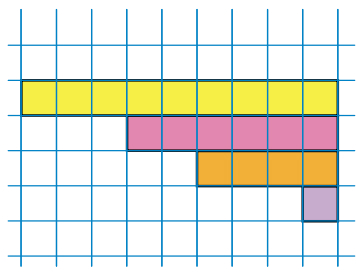Arithmetic progression

## Objective:

To verify that the given sequence is an arithmetic progression.

## Theory:

An arithmetic progression (AP) or arithmetic sequence is a sequence of numbers such that the difference between the consecutive terms is constant. For instance, the sequence 5, 7, 9, 11, 13, 15 … is an arithmetic progression with common difference of 2.

If the first term of an arithmetic progression is a1 and the common difference of successive members is d, then the nth term of the sequence (an) is given by:

an = a1 + (n - 1)d,

A finite portion of an arithmetic progression is called a finite arithmetic progression and sometimes just called an arithmetic progression. The sum of a finite arithmetic progression is called an arithmetic series.

### Examples:-

1- Sequence of 1,4,7,10.......( This is an AP)2- Sequence of 1,4,6,9........( This is not an AP)